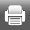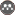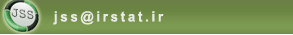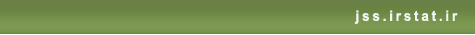[Home ] [Archive]   [ فارسی ]Volume 14, Issue 2 (2-2021)J. of Stat. Sci. 2021, 14(2): 505-534 Back to browse issues page
Bayesian Estimation of Stress-Strength Parameter under Progressive Hybrid Censored Sample in Lomax Distribution
Abstract:   (415 Views)
The Bayesian estimation of the stress-strength parameter in Lomax distribution under the progressive hybrid censored sample is considered in three cases. First, assuming the stress and strength are two random variables with a common scale and different shape parameters. The Bayesian estimations of these parameters are approximated by Lindley method and the Gibbs algorithm. Second, assuming the scale parameter is known, the exact Bayes estimation of the stress-strength parameter is obtained. Third, assuming all parameters are unknown, the Bayesian estimation of the stress-strength parameter is derived via the Gibbs algorithm. Also, the maximum likelihood estimations are calculated, and the usefulness of the Bayesian estimations is confirmed, in comparison with them. Finally, the different methods are evaluated utilizing the Monte Carlo simulation and one real data set is analyzed.
Keywords: Stress-Strength Parameter, Lindley's Approximation, Lomax Distribution, Progressive Hybrid Censored.
Type of Study: Research | Subject: Reliability
Received: 2019/06/22 | Accepted: 2020/06/30 | Published: 2021/02/28
Send email to the article author